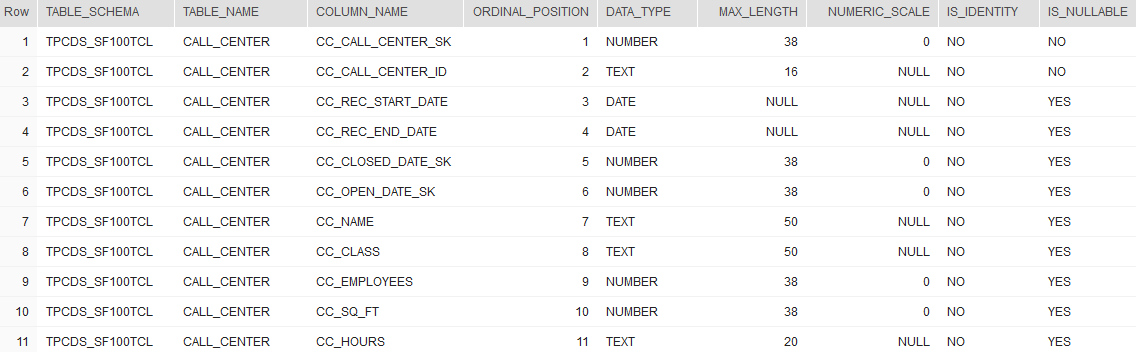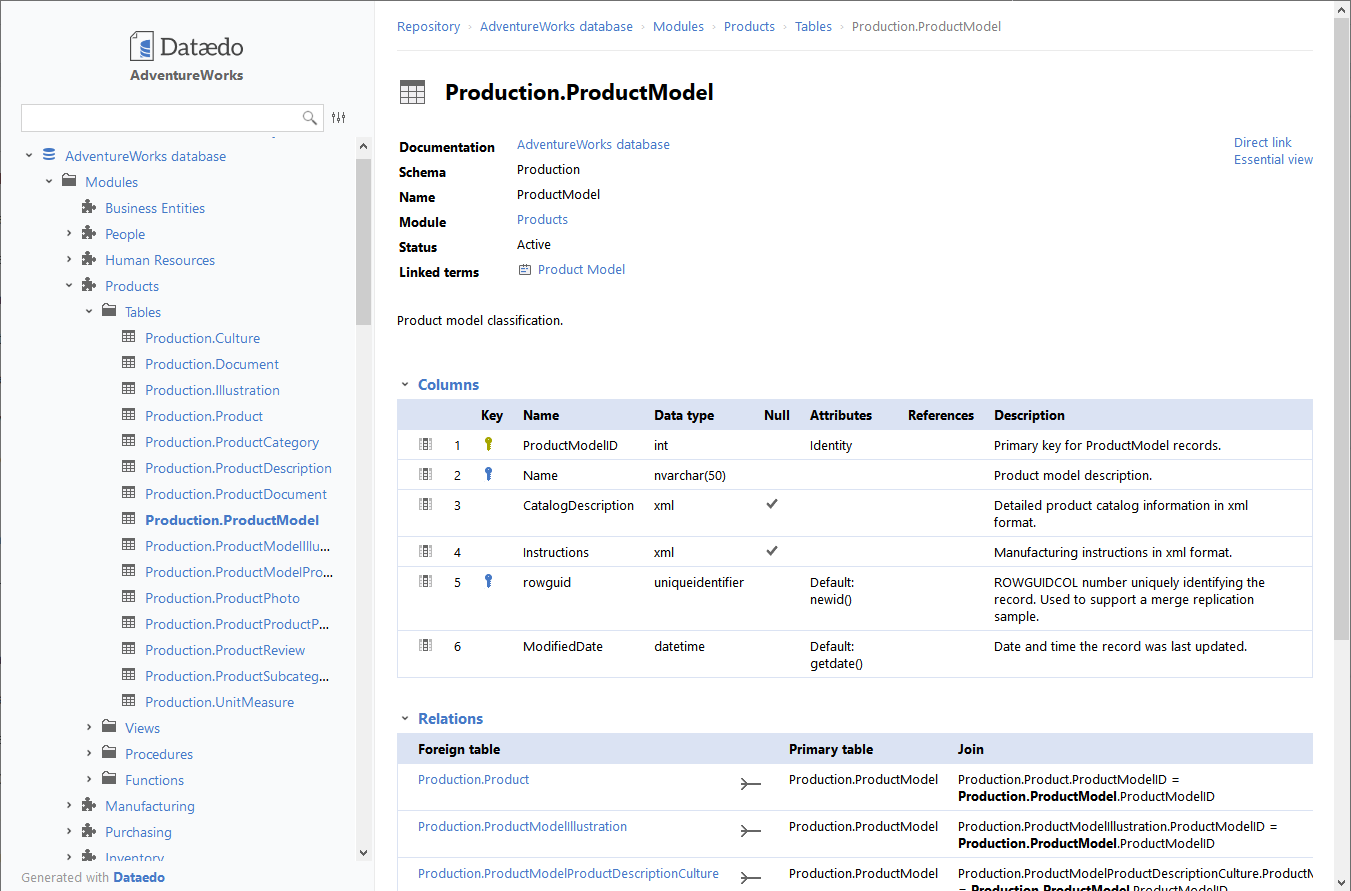## List table columns in Snowflake

Article for:

Query below lists all table columns in a database.

## Query

``````select t.table_schema,
t.table_name,
c.column_name,
c.ordinal_position,
c.data_type,
case
when c.numeric_precision is not null
then c.numeric_precision
when c.character_maximum_length is not null
then c.character_maximum_length
end as max_length,
c.numeric_scale,
c.is_identity,
c.is_nullable
from information_schema.tables t
inner join information_schema.columns c on
c.table_schema = t.table_schema and c.table_name = t.table_name
where table_type = 'BASE TABLE'
order by table_schema,
table_name,
ordinal_position;
``````

## Columns

• table_schema - schema name
• table_name - table name
• column_name - column name
• ordinal_position - number of this column in the table
• data_type - name of the data typ
• max_length - maximum length of the data
• numeric_scale - scale of numeric columns
• description - description of column
• is_identity - identity attribute for the column
• is_nullable - nullability attribute for the column

## Rows

• One row represents one table column
• Scope of rows: all columns in all tables in a database
• Ordered by schema, table name, ordinal_position

## Sample results## You could also get this

Get this interactive HTML data dictionary in minutes with Dataedo.See live HTML data dictionary sample